# Centralisation Aide aux devoirs

## Recommended Posts

C'est bel est bien x^4-4x+1/4

• Replies 755
• Created

#### Popular Days

Oui, avec x², il aurait pu poser X = x², et l'affaire était dans le sac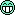##### Share on other sites

owned : t'es en quelle classe?

##### Share on other sites

Et elles sort d'où cette équation ?

##### Share on other sites

En première S mais la prof c'est une folle parfois, c'est un dm que je dois faire

##### Share on other sites

Demande à maple ou mathematica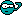##### Share on other sites

Pour une 1er S ca va :)

T'as de la chance avec ton équation car le terme en x^3 est nul et ton équation est déjà normalisée (le terme du degré supérieur vaut 1).

Moi je résouds les équations de dégré 4 avec la méthode de Ferrari mais je sais pas si c'est ce que ta prof attend vue que vous ne l'avez pas vu je pense...

##### Share on other sites

Je préfère la méthode de Lamborghini, bien plus rapide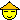(Comme si personne allait la sortir celle là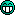)

##### Share on other sites

1ère S, c'est bien le mini pour ce type d'équation...

un petit collage d'une recherche sur le net

désolé mais même prof, j'ai jamais eû à résoudre ce type d'équation

on m'a plutôt fait travaillé sur des maths intéressantes...

c'est vraiment le genre de travail débile que je suis bien content d'avoir évité...bien le bonjour à ta prof.

Polynômes est degré 4

On part de l'équation x^4 + a * x^3 + b * x^2 + c * x + d = 0.

On effectue le changement de variable x = z - a/4.

On obtient une équation réduite de la forme:

z^4 + p * z^2 + q * z + r = 0

Avec p = b - (3/:chinois:*a^2 ;

q = c - a*b/2 + (1/:D*a^3 et

r = d - a*c/4 + (1/16)*b*a^2 - (3/256)*a^4

On a deux cas pour l'équation en z: (i) q = 0 et (ii) q <>0.

(i) q = 0. L'équation s'écrit z^4 + p * z^2 + r = 0.

C'est ce que l'on appelle une équation bicarrée.

On pose y = z^2 et l'equation devient y^2 + p * y + r = 0

Les solutions sont donc:

y1 = -p/2 + sqrt( (1/4)*p^2 - r) et y2 = -p/2 - sqrt( (1/4)*p^2 - r)

De là les valeurs de z sont:

z1 = sqrt(y1) ; z2 = - sqrt(y1) ; z3 = sqrt(y2) et z4 = -sqrt(y2).

(ii) q <> 0. L'équation s'écrit z^4 + p * z^2 + q * z + r = 0

On pose alors 2*P - Q^2 = p ; -2*Q*R = q et P^2 - R^2 = r.

On a alors (z^2 + P)^2 - (Qz +R)^2 = 0. Ce qui est une autre façon

d'écrire z^4 + p * z^2 + q * z + r = 0.

Si l'on arrive à determiner le triplet (P0, Q0, R0) alors trouver les

solutions de l'équation réduite revient à résoudre:

z^2 + P0 + Q0 * z + R0 = 0 ou

z^2 + P0 - Q0 * z - R0 = 0

On peut donc trouver z.

Il reste donc à determiner P, Q et R. C'est à dire à résoudre le système

{ 2*P - Q^2 = p

{ -2*Q*R = q

{ P^2 - R^2 = r

Ce système revient à:

{ Q^2 = q^2 / (4*(P^2 - r))

{ R^2 = P^2 - r

{ Q*R = -q / 2

Ce qui revient à résoudre l'équation (en P) suivante:

P^3 - (p/2)*P^2 - r*P + p*r/2 - (1/8)*q^2 = 0

De là, on trouve (pas si) facilement P0. Et grâce au système on peut

lui associer un couple (Q0, R0) et donc trouver z... (ouf !)

si il y en a un qui trouve cela intéressant, qu'il branche la prof de ownedkiller, ça lui fera du bienEdited by noisette
##### Share on other sites

Apupadi a trouver sa sur le net :

Etape n°1 : normalisation de l'équation :

1x^4-4x+1/4 = 0

Etape n°2 : changement de variable : x = X + 0

le terme en x^3 disparaît

1x^4-4x+1/4 = 0

Cette équation est équivalente à :

X^4 + X²t + 1/4 t² =

( t - 0)X² - -4X + 1/4 t² - 1/4 (E)

Etape n°3 :

Le second membre de cette équation est un carré parfait

si le discriminant du polynôme du second membre est nul,

ce qui se produit si t est solution de l'équation :

1t^3-t-16 = 0

Une solution réelle de cette équation est t0 = 2.65201

Etape n ° 4 : résolution de l'équation en X

X1 = 1.5659954794881241

X2 = 0.06250382969195667

X3 = -0.8142496545900404+1.3751824552588288i

X4 = -0.8142496545900404-1.3751824552588288i

Etape n°5 : En tenant compte du premier changement de variable on a :

x1 = 1.5659954794881241

x2 = 0.06250382969195667

x3 = -0.8142496545900404+1.3751824552588288i

Mais je ne comprend pas d'ou sort le t

##### Share on other sites

Je confirme le résultat ( obtenu par Matlab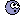) à ceci près qu'il y a aussi le conjugé de x3 en plusPar contre sur la méthode : aucune idée de comment faire. Faut être barré pour donné ca à des 1ere S.

T'es sur que c'est bien ca, l'équation, je veux dire que la prof s'est peut être gourré. Quelqu'un a demandé dans la classe ? Pour moi, vous devriez tombé sur des solutions évidentes, pas des nombres de barbares: personne ne s'amuse à résoudre ca à la main, à part les matheux mazo.

PS: les valeurs exacte ca donne ca: 1/6*3^(1/2)*(((216+3*5181^(1/2))^(2/3)+3)/(216+3*5181^(1/2))^(1/3))^(1/2)+1/6*((-3*(((216+3*5181^(1/2))^(2/3)+3)/(216+3*5181^(1/2))^(1/3))^(1/2)*(216+3*5181^(1/2))^(2/3)-

9*(((216+3*5181^(1/2))^(2/3)+3)/(216+3*5181^(1/2))^(1/3))^(1/2)+72*3^(1/2)*(216+3*5181^(1/2))^(1/3))/(216+3*5181^(1/2))^(1/3)/(((216+3*5181^(1/2))^(2/3)+3)/(216+3*5181^(1/2))^(1/3))^(1/2))^(1/2

1/6*3^(1/2)*(((216+3*5181^(1/2))^(2/3)+3)/(216+3*5181^(1/2))^(1/3))^(1/2)-1/6*((-3*(((216+3*5181^(1/2))^(2/3)+3)/(216+3*5181^(1/2))^(1/3))^(1/2)*(216+3*5181^(1/2))^(2/3)-

9*(((216+3*5181^(1/2))^(2/3)+3)/(216+3*5181^(1/2))^(1/3))^(1/2)+72*3^(1/2)*(216+3*5181^(1/2))^(1/3))/(216+3*5181^(1/2))^(1/3)/(((216+3*5181^(1/2))^(2/3)+3)/(216+3*5181^(1/2))^(1/3))^(1/2))^(1/2)

-1/6*3^(1/2)*(((216+3*5181^(1/2))^(2/3)+3)/(216+3*5181^(1/2))^(1/3))^(1/2)+1/6*i*((3*(((216+3*5181^(1/2))^(2/3)+3)/(216+3*5181^(1/2))^(1/3))^(1/2)*(216+3*5181^(1/2))^(2/3)+

9*(((216+3*5181^(1/2))^(2/3)+3)/(216+3*5181^(1/2))^(1/3))^(1/2)+72*3^(1/2)*(216+3*5181^(1/2))^(1/3))/(216+3*5181^(1/2))^(1/3)/(((216+3*5181^(1/2))^(2/3)+3)/(216+3*5181^(1/2))^(1/3))^(1/2))^(1/2)

-1/6*3^(1/2)*(((216+3*5181^(1/2))^(2/3)+3)/(216+3*5181^(1/2))^(1/3))^(1/2)-1/6*i*((3*(((216+3*5181^(1/2))^(2/3)+3)/(216+3*5181^(1/2))^(1/3))^(1/2)*(216+3*5181^(1/2))^(2/3)+

9*(((216+3*5181^(1/2))^(2/3)+3)/(216+3*5181^(1/2))^(1/3))^(1/2)+72*3^(1/2)*(216+3*5181^(1/2))^(1/3))/(216+3*5181^(1/2))^(1/3)/(((216+3*5181^(1/2))^(2/3)+3)/(216+3*5181^(1/2))^(1/3))^(1/2))^(1/2)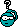##### Share on other sites

Pile poil ce que j'avais trouvé en essayant de tête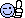##### Share on other sites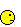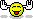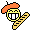##### Share on other sites
• 5 months later...

Bien le bonjour ^^

Alors voila, cela peut paraitre con me direz vous, mais j'ai un problème pour calculer la limite de quelquechose d'assez simple en somme.

En fait je dois calculer le nombre dérivée de -1, sur la fonction x²-1+x. Je ne peux calculer la dérivée pour y arriver, je dois OBLIGATOIREMENT passer par la limite.

pouvez vous m'aidez à le calculer ?

Merci ^^

##### Share on other sites

Bien le bonjour ^^

Alors voila, cela peut paraitre con me direz vous, mais j'ai un problème pour calculer la limite de quelquechose d'assez simple en somme.

En fait je dois calculer le nombre dérivée de -1, sur la fonction x²-1+x. Je ne peux calculer la dérivée pour y arriver, je dois OBLIGATOIREMENT passer par la limite.

pouvez vous m'aidez à le calculer ?

Merci ^^

si j'ai bien compris, tu cherches f'(-1) pur f(x) = x²-1+x, qui est définie et dérivable en -1 :

On part du fait que f'(-1) := lim (x tend vers -1) de ((f(x) - f(-1))/(x-(-1))

écris-le quelque part : qu'est-ce que ça donne ?

tu dois avoir ça:

f'(-1) = lim (x tend vers -1) de (x²-1+x +1)/x+1

regroupe et simplifie :

tu trouves quoi ?

normalement , çà :

f'(-1) = lim (x tend vers -1) de (x²+x )/x+1

suivi de ça :

f'(-1) = lim (x tend vers -1) de x

donc c'est égal à :

=-1.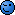##### Share on other sites

Roooo noisette qui fait tout le boulot.

Je savais pas que les profs ils donnaient directement les réponses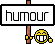##### Share on other sites

Rien compris##### Share on other sites

http://www.pcinpact.com/forum/index.php?showtopic=61487

--tousss--##### Share on other sites

Roooo noisette qui fait tout le boulot.

Je savais pas que les profs ils donnaient directement les réponsesj'ai laissé des blancsje le referai plus c'est promisen même temps, c'est une des faiblesses des programmes d'aujourd'hui de ne pas détailler la définition des dérivées et c'est tout à l(honneur de son prof de faire réfléchir là-dessus sur un cas simple ()

Rien compris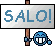http://www.pcinpact.com/forum/index.php?showtopic=61487

--tousss--ahem, c'est vrai, il y a un topic d'aide aux devoirs...

voir le lien de Keneda.##### Share on other sites

Sinon... Pour le français : Pouvez-vous m'aider pour mes maths ?

Voire même "pourriez-vous", ça rend mieux##### Share on other sites

Bonjour à tous,

Je suis sur une équation de lycée que je n'arrive pas à résoudre ='(Pouvez-vous m'aider ? Merci !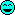##### Share on other sites

Elle est où l'equation ?

Ce n'est qu'un simple resultat là...

##### Share on other sites

Comme l'a dit noisette dans l'autre topic, si tu veux une réponse qui ne soit pas sous forme de quotient ça donne en toute logique:

10^17 kg/m^3

##### Share on other sites

Bonsoir ! Bon alors j'ai un problème en électricité. Je suis en première et n'ayant jamais vraiment toucher l'élec je suis un peu perdu. J'ai une table de verité à établir d'un schéma, ce que j'ai fait d'après les methodes du prof mais j'ai pas l'impression que je soit très juste si quelqu'un peut verifier ?Merci d'avance...

##### Share on other sites

Tu es en première quoi?